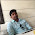### Use of Intersheath for Grading

In this method of grading, in between the core and the lead sheath number of metallic sheaths are placed which are called intersheaths. All these intersheaths are maintained at different potentials by connecting them to the tappings of the transformer secondary. These potentials are between the core potential and earth potential. Generally lead is used for these sheaths as it is flexible and corrosion resistance but as its mechanical strength is less, aluminium also can be used. Aluminium is low weight and mechanically strong but it is much costlier than lead.
Using the intersheaths, maintaining at different potential, uniform distribution of stress is obtained in the cables.
Consider a cable with core diameter d and overall diameter with lead sheath as D. Let two intersheaths are used having diameter d1 and d2 which are kept at the potentials V1 and V2 respectively.
The intersheaths and stress distribution is shown in the Fig. 1.Fig. 1

Let    V1 = Voltage of intersheath 1 with respect to earth
V2 = Voltage of intersheath 2 with respect to earth
It has been proved that stress at a point which is at a distance x is inversely proportional to distance x and given by,

Where k is constant.
So electric stress between he conductor and intersheath 1 is,
Now potential difference between core and the first intersheath is V-V1.
Substituting in equation (2) we get,
Now this stress is maximum at x = d/2, on core surface.
Similarly potential difference between intersheath 1 and intersheath 2 is V1 - V2.
Now g1 will be maximum at the surface of intersheath 1 i.e. x = d1/2.
The potential difference between intersheath 2 and outermost sheath V2 is only as potential of intersheath is maintained at V2 with respect to earth.
This g3 will be maximum at x = d2/2
Choosing proper values of V1 and V2, g1max, g2max etc. can be made equal and hence uniform distribution of stress can be obtained.
The stress can be made to vary between same maximum and minimum values as shown in the Fig. 1, by choosing d1 and d2 such that,
d1/d = d2/d1 = D/d2 = α
and    g1max = g2max = g3max

Let us try the express voltages V1 and V2 interms of V and α.
Substituting value of V2 from equation (11),

1.2.